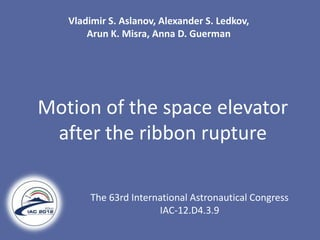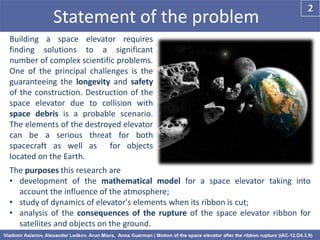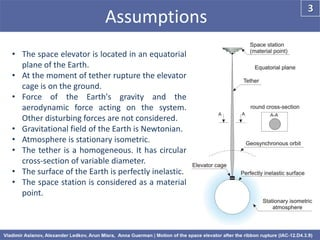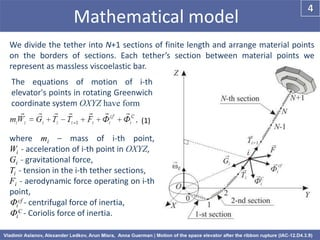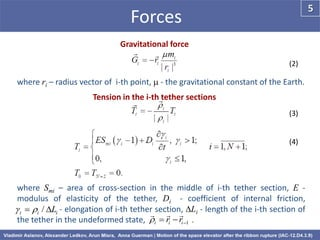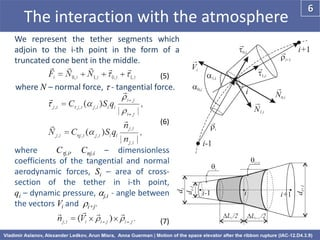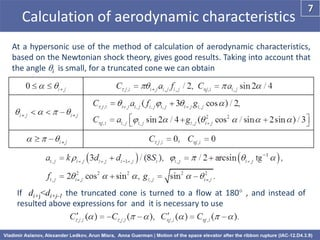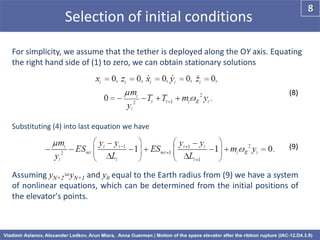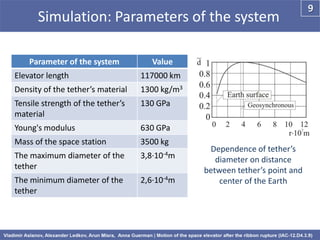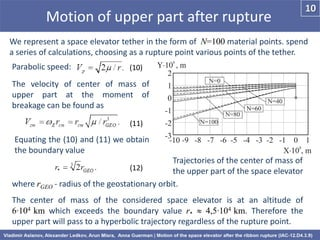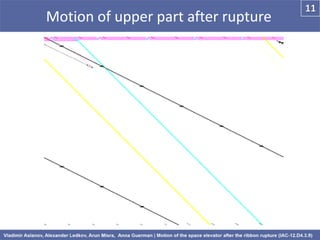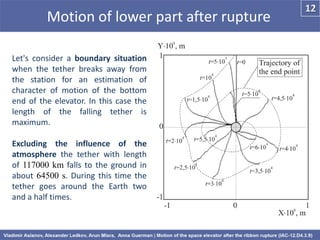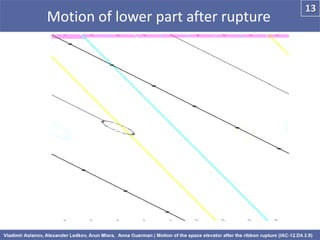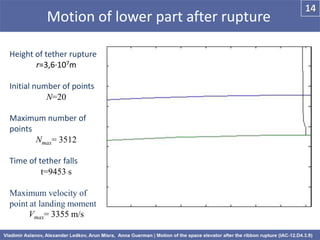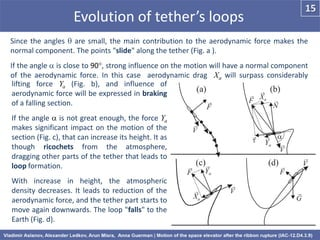1 of 15

### Motion of the space elevator after the ribbon rupture

1. Vladimir S. Aslanov, Alexander S. Ledkov, Arun K. Misra, Anna D. Guerman Motion of the space elevator after the ribbon rupture The 63rd International Astronautical Congress IAC-12.D4.3.9
2. 2 Statement of the problem Building a space elevator requires finding solutions to a significant number of complex scientific problems. One of the principal challenges is the guaranteeing the longevity and safety of the construction. Destruction of the space elevator due to collision with space debris is a probable scenario. The elements of the destroyed elevator can be a serious threat for both spacecraft as well as for objects located on the Earth. The purposes this research are • development of the mathematical model for a space elevator taking into account the influence of the atmosphere; • study of dynamics of elevator's elements when its ribbon is cut; • analysis of the consequences of the rupture of the space elevator ribbon for satellites and objects on the ground.
3. 3 Assumptions • The space elevator is located in an equatorial plane of the Earth. • At the moment of tether rupture the elevator cage is on the ground. • Force of the Earth's gravity and the aerodynamic force acting on the system. Other disturbing forces are not considered. • Gravitational field of the Earth is Newtonian. • Atmosphere is stationary isometric. • The tether is a homogeneous. It has circular cross-section of variable diameter. • The surface of the Earth is perfectly inelastic. • The space station is considered as a material point.
4. 4 Mathematical model We divide the tether into N+1 sections of finite length and arrange material points on the borders of sections. Each tether’s section between material points we represent as massless viscoelastic bar. The equations of motion of i-th elevator's points in rotating Greenwich coordinate system OXYZ have form (1) where mi – mass of i-th point, Wi - acceleration of i-th point in OXYZ, Gi - gravitational force, Ti - tension in the i-th tether sections, Fi - aerodynamic force operating on i-th point, icf - centrifugal force of inertia, iС - Coriolis force of inertia.
5. 5 Forces Gravitational force (2) where ri – radius vector of i-th point,  - the gravitational constant of the Earth. Tension in the i-th tether sections (3) (4) where Smi – area of cross-section in the middle of i-th tether section, E - modulus of elasticity of the tether, Di - coefficient of internal friction, _________i - elongation of i-th tether section, DLi - length of the i-th section of i  i / DL   i  ri  ri 1 the tether in the undeformed state, __________.
6. 6 The interaction with the atmosphere We represent the tether segments which adjoin to the i-th point in the form of a truncated cone bent in the middle. (5) where N – normal force,  - tangential force. (6) where Cj,i, Cnj,i – dimensionless coefficients of the tangential and normal aerodynamic forces, Si – area of cross- section of the tether in i-th point, qi – dynamic pressure, j,i - angle between the vectors Vi and i+j. (7)
7. 7 Calculation of aerodynamic characteristics At a hypersonic use of the method of calculation of aerodynamic characteristics, based on the Newtonian shock theory, gives good results. Taking into account that the angle i is small, for a truncated cone we can obtain If di+j<di+j-1 the truncated cone is turned to a flow at 180 , and instead of resulted above expressions for and it is necessary to use
8. 8 Selection of initial conditions For simplicity, we assume that the tether is deployed along the OY axis. Equating the right hand side of (1) to zero, we can obtain stationary solutions (8) Substituting (4) into last equation we have (9) Assuming yN+2=yN+1 and y0 equal to the Earth radius from (9) we have a system of nonlinear equations, which can be determined from the initial positions of the elevator's points.
9. 9 Simulation: Parameters of the system Parameter of the system Value Elevator length 117000 km Density of the tether’s material 1300 kg/m3 Tensile strength of the tether’s 130 GPa material Young's modulus 630 GPa Mass of the space station 3500 kg Dependence of tether’s The maximum diameter of the 3,8∙10-4m diameter on distance tether between tether’s point and The minimum diameter of the 2,6∙10-4m center of the Earth tether
10. 10 Motion of upper part after rupture We represent a space elevator tether in the form of N=100 material points. spend a series of calculations, choosing as a rupture point various points of the tether. Parabolic speed: (10) The velocity of center of mass of upper part at the moment of breakage can be found as (11) Equating the (10) and (11) we obtain the boundary value Trajectories of the center of mass of (12) the upper part of the space elevator where rGEO - radius of the geostationary orbit. The center of mass of the considered space elevator is at an altitude of 6∙104_km which exceeds the boundary value r*  4,5∙104 km. Therefore the upper part will pass to a hyperbolic trajectory regardless of the rupture point.
11. 12 Motion of lower part after rupture Let's consider a boundary situation when the tether breaks away from the station for an estimation of character of motion of the bottom end of the elevator. In this case the length of the falling tether is maximum. Excluding the influence of the atmosphere the tether with length of 117000 km falls to the ground in about 64500 s. During this time the tether goes around the Earth two and a half times.
12. 14 Motion of lower part after rupture Height of tether rupture r=3,6∙107m Initial number of points N=20 Maximum number of points Nmax= 3512 Time of tether falls t=9453 s Maximum velocity of point at landing moment Vmax= 3355 m/s
13. 15 Evolution of tether’s loops Since the angles  are small, the main contribution to the aerodynamic force makes the normal component. The points "slide" along the tether (Fig. a ). If the angle  is close to 90, strong influence on the motion will have a normal component of the aerodynamic force. In this case aerodynamic drag Xa will surpass considerably lifting force Ya (Fig. b), and influence of aerodynamic force will be expressed in braking of a falling section. If the angle  is not great enough, the force Ya makes significant impact on the motion of the section (Fig. c), that can increase its height. It as though ricochets from the atmosphere, dragging other parts of the tether that leads to loop formation. With increase in height, the atmospheric density decreases. It leads to reduction of the aerodynamic force, and the tether part starts to move again downwards. The loop "falls" to the Earth (Fig. d).
14. 16 Conclusion The problem of investigation of dynamics of the space elevator after its destruction by space debris was considered. A mathematical model, in which the flexible heavy tether of circular cross-section is represented as a set of the mass points connected by a set of massless viscoelastic bars was developed. A distinctive feature of the model is the consideration of aerodynamic forces acting on the tether. The results of the simulation show that • The upper section of the space elevator will pass to a hyperbolic trajectory regardless of the rupture point. • Rupture of the space elevator ribbon can jeopardize the spacecraft in the equatorial plane since the ribbon moves with rather large velocity. • The lower part of the space elevator after the ribbon enters the atmosphere. Most of it slows down and falls smoothly, but some elements reach the Earth surface with rather large velocities. These parts of the tether can put in danger objects on the ground.
15. 17 References 1. Aslanov, V.S., Ledkov, A.S., Dynamics of tethered satellite systems, Woodhead Publishing, Cambridge, 2012. 2. Pearson, J., "The Orbital Tower: A Spacecraft Launcher Using the Earth's Rotational Energy", Acta Astronautica, №2, 1975, pp. 785-799. 3. Smitherman, D.V. Jr., "Space Elevator: An Advanced Earth-Space Infrastructure for the New Millenium", Marshall Space Flight Center. Huntsville, Alabama. NASA/CP-2000-210429. August 2000. 4. Edwards, B.C., "Design and deployment of a space elevator", Acta Astronautica, №2, 2000, pp. 785–799. 5. Sadov, Yu.A., Nuralieva, A.B. "On Conception of the Loaded Sectioned Space Elevator", Preprint of Keldysh Institute of Applied Mathematics RAS. Moscow, 2011. 6. Edwards, B.C., "The Space Elevator", Final Report on the theme "The Space Elevator" for the NASA Institute for Advanced Concepts (NIAC). 80 p. 7. Quine, B.M., Seth, R.K., Zhu, Z.H., "A free-standing space elevator structure: A practical alternative to the space tether", Acta Astronautica, №65, 2009, pp. 365-375. 9. Perek, L., " Space elevator: Stability“, Acta Astronautica, Vol.62, №8–9, 2008, p. 514–520. 10. Alpatov, A.P., Dranovskii, V.I., Zakrzhevskii, A.E., Pirozhenko, A.V., "Space tether systems. The problem review", Cosmic Science and Technology, Vol.3, №5/6, 1997, pp. 21-29. (in Russian) 11. Aslanov, V.S., Ledkov, A.S., Stratilatov, N.R., "Spatial Motion of Space Rope Cago Transport System", Scientific and technical journal "Polyot" ("Flight"), №2, 2007, pp. 28-33. (in Russian) 12. Williams, P., "Dynamic multibody modeling for tethered space elevators", Acta Astronautica, №65, 2009, pp. 399–422. 13. Beletsky, V.V., Levin, E.M., "Dynamics of space tether systems", Univelt, Inc., San Diego, 1993, p. 499. 14. Arjannikov, N.S., Sadekova, G.S., "Aerodynamics of a flying vehicle", Higher School, Moscow, 1983.